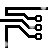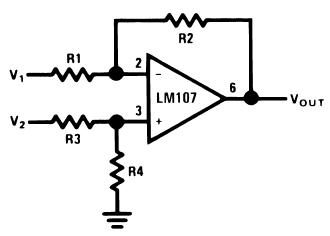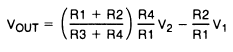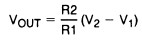## The Difference Amplifier

### By internum

The difference amplifier is the complement of the summing amplifier and allows the subtraction of two voltages or, as a special case, the cancellation of a signal common to the two inputs.

The difference amplifier is the complement of the summing amplifier and allows the subtraction of two voltages or, as a special case, the cancellation of a signal common to the two inputs. This circuit is shown in Figure 1 and is useful as a computational amplifier, in making a differential to single-ended conversion or in rejecting a common mode signal.Figure 1. Difference AmplifierFor R1 = R3 and R2 = R4R1 || R2 = R3 || R4
For minimum offset error due to input bias current

Circuit bandwidth may be calculated in the same manner as for the inverting amplifier, but input impedance is somewhat more complicated. Input impedance for the two inputs is not necessarily equal; inverting input impedance is the same as for the inverting amplifier of Figure 1 and the non-inverting input impedance is the sum of R3 and R4. Gain for either input is the ratio of R1 to R2 for the special case of a differential input single-ended output where R1 = R3 and R2 = R4. The general expression for gain is given in the figure. Compensation should be chosen on the basis of amplifier bandwidth.

Care must be exercised in applying this circuit since input impedances are not equal for minimum bias current error.198109 December 2007